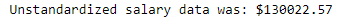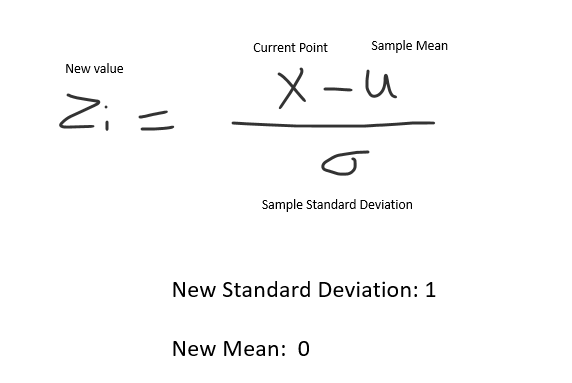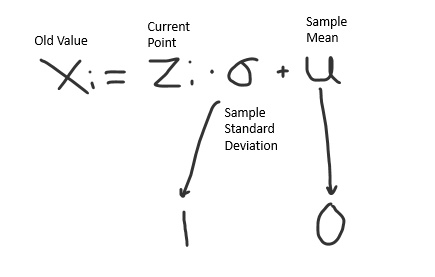# Machine Learning 101: Reverse Standardization

We’ve all been there; you’ve worked night and day to finally get an accurate model for your dataset. You’ve finally got an output from your model – but it’s scaled. What do you do? How do you reverse standardization?

In this 2-minute guide, we’ll go over how you can find out the real target value for your model prediction and how you can reverse it.

If you’re here for a quick code chunk for a model prediction, here is a python function to get you on your way.

## Reverse Standardization In Python For Model Prediction

``````import pandas as pd
import numpy as np

# example data

# lets say your model gave you an output
# for a salary
# here's how you can reverse engineer it from
# the target column
def reverse_standardization_pred(col, prediction):

# calculate the mean
mean = sum(col) / len(col)

# calculate the variance
var = sum((val - mean)**2 for val in col) / len(col)

# calculate standard deviation
std = var ** 0.5

# apply it to the prediction
real_val = prediction * std + mean

return real_val

# your model predicted a salary, how to reverse it
# where the .25 is your **models** prediction
real_salary = reverse_standardization(df['salary_in_usd'], .25)

print(f'Unstandardized salary data was: \${round(real_salary,2)}')``````## Why You Should Standardize Variables

Standardizing variables is important in order to ensure that the results of statistical tests are accurate and meaningful.

When variables are not standardized, it can be difficult to determine whether the results of a test are statistically significant.

Some machine learning models, like lasso and ridge regression, depend on scaled data.

Models that utilize gradient descent have shown improvements when data is standardized.

## Why we can’t rebuild a dataset from standardized data (Without the Old Data)

We can’t rebuild a dataset from standardized data without the old data because of how standardization is done in the first place.

Let’s take a look.

The formula for standardization is the following (for each data point):Once we’ve applied this transformation to our data, we now have a standardized column with a mean at zero and a standard deviation of one.

If we wanted to reverse engineer this column (without the old data), the formula would be the following.Using this formula, every point maps to itself since we multiply it by 1 and add 0.

This is why (without the old standard deviation and mean) we cannot reverse standardize the data.

## Other Articles in our Machine Learning 101 Series

We have many quick guides that go over some of the fundamental parts of machine learning. Some of those guides include: